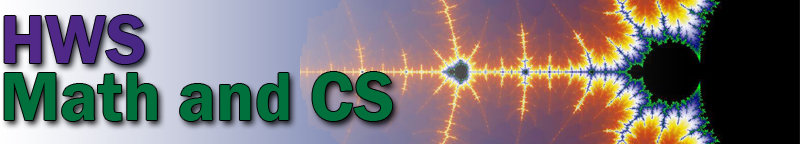Schedule of Courses for Fall 2019 Period 1 MWF 8:30–9:30 Math 130: Calculus I, Yan Hao       (Lab Tuesday, 12:15–1:45) Math 232: Multivariable Calculus, Alden Gassert Period 1B MWF 9:45–10:45 Math 130: Calculus I, William Simmons       (Lab Tuesday, 8:45–10:15) Math 131: Calculus II, Erika King       (Lab Thursday 8:45–10:15) CPSC 371: Programming Languages, John Lasseter Period 2 MWF 11:00–12 Math 130: Calculus I, William Simmons       (Lab Tuesday, 10:305–12:00) Math 131: Calculus II, Erika King       (Lab Thursday 10:30–12:00) Math 135: First Steps into Advanced Math, David Eck CPSC 225: Intermediate Programming, Chris Fietkiewicz       (Lab Thursday, 10:30–12:00) CPSC 329: Software Development, Stina Bridgeman       (Lab Tuesday, 10:30–12:00) Period 3 MWF 12:15–1:15 Math 130: Calculus I, Jocelyn Bell       (Lab Tuesday, 2:005–3:30) Math 214: Applied Linear Algebra, Yan Hao CPSC 124: Introductory Programming, John Lasseter       (Lab Thursday, 12:15–1:45) CPSC 424: Computer Graphics, Stina Bridgeman       (Lab Tuesday, 12:15–1:45) Period 4 MWF 1:30–2:30 Math 331: Foundations of Analysis, David Eck Math 100: Elementary Functions, Alden Gassert       (Lab Thursday,12:15–1:45) CPSC 124: Introductory Programming, John Lasseter       (Lab Thursday, 2:00–3:30) Period 4A MW 1:30–3:00 Math 204: Linear Algebra, Jocelyn Bell Period 9 TuTh 2:00–3:30 Math 110: Discovering in Mathematics, Erika King Math 353: Mathematical Modeling, Jon Forde CPSC 220: Computer Architecture, Chris Fietkiewicz       (Lab Friday, 1:30–3:00) Period 10 TuTh 3:45–5:15 Math 114: Mathemathics for Informed Citizenship, Jon Forde

 Tentative Schedule of Courses for Spring 2020 Period 1 MWF 8:30–9:30 Math 130: Calculus I, David Eck       (Lab Tuesday, 12:15–1:45) CPSC 124: Introductory Programming, Chris Fietkiewicz       (Lab Thursday, 8:45–10:15) Period 1B MWF 9:45–10:45 Math 130: Calculus I, Alden Gassert       (Lab Tuesday, 8:45–10:15) Math 232: Multivariable Calculus, William Simmons Math 100: Elementary Functions, Yan Hao       (Lab Thursday, 12:15–1:45) CPSC 433: Compilers, John Lasseter       (Lab Tuesday, 2:00–3:30) Period 2 MWF 11:00–12 Math 130: Calculus I, Alden Gassert       (Lab Tuesday, 8:45–10:15) Math 204: Linear Algebra, David Eck Math 375: Abstract Algebra, Jennifer Biermann CPSC 225: Programming II, Stina Bridgeman       (Lab Thursday, 2:00–3:30) Period 3 MWF 12:15–1:15 Math 131: Calculus II, William Simmons       (Lab Thurday, 8:45–10:15) Math 350: Probability, Yan Hao CPSC 229: Foundations of Computation, John Lasseter Period 4 MWF 1:30–2:30 Math 130: Calculus I, Jennifer Biermann       (Lab Tuesday, 2:00–3:30) Math 131: Calculus II, Erika King       (Lab Thursday, 10:30–12:00) CPSC 327: Data Structures and Algorithms, Stina Bridgeman Period 5 MW 3:15—4:45 Math 237: Differential Equations, Jon Forde Period 6 TuTh 8:45–10:15 Math 471: Mathematics Capstone, Joseph Rusinko Period 7 TuTh 10:30–12:00 CPSC 331: Operating Systems, Chris Fietkiewitz Period 9 TuTh 2:00–3:30 Math 135: First Steps into Advanced Math, Erika King Period 10 TuTh 3:45–5:15 Math 114: Mathemathics for Informed Citizenship, Jon Forde